# The parabolic segment

The parabolic segment has a base a = 4 cm and a height v = 6 cm. Calculate the volume of the body that results from the rotation of this segment

a) around its base
b) around its axis.

Result

V1 =  0 cm3
V2 =  50.265 cm3

#### Solution:

$a = 4 \ cm \ \\ v = 6 \ cm \ \\ f(x) = q x^2 \ \\ f(a/2) = q (a/2)^2 = v \ \\ 6 = q \cdot \ 2^2 \ \\ \ \\ V_{1}=q = 6/2^2 = \dfrac{ 3 }{ 2 } = 1.5 = 0 \ cm^3$
$f(x) = v - \dfrac{ 6 }{ 4 } x^2 \ \\ f(x) = 6 - \dfrac{ 6 }{ 4 } x^2 \ \\ \ \\ x_{ 0 } = -a/2 = -4/2 = -2 \ \\ x_{ 1 } = a/2 = 4/2 = 2 \ \\ \ \\ V_{ 2 } = \pi \cdot \ \int_\{ x_{ 0 } \}^\{x_{ 1 }\} f(x) dx \ \\ V_{ 2 } = \pi \cdot \ \int_\{x_{ 0 }\}^\{x_{ 1 }\} (\dfrac{ 6 }{ 4 } x^2 - v) dx \ \\ V_{ 2 } = \pi \cdot \ [\dfrac{ 6 }{ 4 } \cdot \ x^3/3 - vx]_\{x_{ 0 }\}^\{x_{ 1 }\} \ \\ \ \\ F(x) = 6x - \dfrac{ 6 }{ 4 } \cdot \ x^3/3 \ \\ F_{ 1 } = 6 \cdot \ x_{ 1 } - \dfrac{ 6 }{ 4 } \cdot \ x_{ 1 }^3/3 = 6 \cdot \ 2 - \dfrac{ 6 }{ 4 } \cdot \ 2^3/3 = 8 \ \\ F_{ 0 } = 6 \cdot \ x_{ 0 } - \dfrac{ 6 }{ 4 } \cdot \ x_{ 0 }^3/3 = 6 \cdot \ (-2) - \dfrac{ 6 }{ 4 } \cdot \ (-2)^3/3 = -8 \ \\ \ \\ V_{ 2 } = \pi \cdot \ (F_{ 1 } - F_{ 0 }) = 3.1416 \cdot \ (8 - (-8)) \doteq 50.2655 = 50.265 \ cm^3$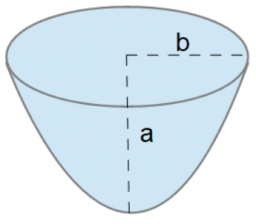Our examples were largely sent or created by pupils and students themselves. Therefore, we would be pleased if you could send us any errors you found, spelling mistakes, or rephasing the example. Thank you!

Leave us a comment of this math problem and its solution (i.e. if it is still somewhat unclear...):Be the first to comment!## Next similar math problems:

1. Reciprocal value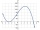How do I calculate a number x that is 9 greater than its reciprocal (1/x)?
2. RectangleCalculate area of the rectangle if its length is 12 cm longer than its width and length is equal to the square of its width.
3. Rectangular garden 2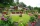A farmer bought 600 m of wire for the fence. He wants to use it to besiege a rectangular garden with a surface of 16875 m2. Calculate the size of the garden.
4. Symmetry by plane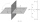Determine the coordinates of a image of point A (3, -4, -6) at a symmetry that is determined by the plane x-y-4z-13 = 0
5. Theorem proveWe want to prove the sentence: If the natural number n is divisible by six, then n is divisible by three. From what assumption we started?
6. Expressions 3If k(x+6)= 4x2 + 20, what is k(10)=?
7. GP membersThe geometric sequence has 10 members. The last two members are 2 and -1. Which member is -1/16?Which of the points belong function f:y= 2x2- 3x + 1 : A(-2, 15) B (3,10) C (1,4)
9. Parametric equationFind the parametric equation of a line with y-intercept (0,-4) and a slope of -2.
10. AsymptoteWhat is the vertical asymptote of ?
11. Third memberDetermine the third member of the AP if a4=93, d=7.5.
12. Normal distribution GPA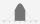The average GPA is 2.78 with a standard deviation of 4.5. What are students in the bottom the 20% having what GPA?Write the equation of the quadratic function which includes points A (-1, 10), B (2, 19), C (1,4)Solve the quadratic equation: m2=4m+20 using completing the square methodThe lengths of a parallel sides of a trapezium are (2x+3) and (x+8) and the distance between them is (x+4). if the area of the trapezium is 590 , find the value of x.Three lorries droved bricks. One drove n bricks at once, second m less bricks than the first and third 300 bricks more the first lorry. The first lorry went 4 times a day the largest went 3 times a day and the smallest 5 times a day. How many bricks brFind the term independent of x in the expansion of (4x3+1/2x)8